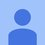# Conversion

Two isomeric alkyl bromides (A) & (B), $$\ce{C5H11Br}$$ yield the following results in the laboratory. (A), on treatment with alcoholic $$\ce{ KOH}$$ gives (C) & (D). $$\ce{C5H10}$$. (C), on Ozonolysis gives HCHO and 2-Methyl propanal. (B) on treatment with alcoholic KOH gives (D) & (E), $$\ce{C5H10}$$. All the compounds (C), (D), (E) on catalytic hydrogenation give (F), $$\ce{C5H12}$$. Deduce the structures from (A) to (F).Note by Rajdeep Bharati
4 years, 9 months ago

This discussion board is a place to discuss our Daily Challenges and the math and science related to those challenges. Explanations are more than just a solution — they should explain the steps and thinking strategies that you used to obtain the solution. Comments should further the discussion of math and science.

When posting on Brilliant:

• Use the emojis to react to an explanation, whether you're congratulating a job well done , or just really confused .
• Ask specific questions about the challenge or the steps in somebody's explanation. Well-posed questions can add a lot to the discussion, but posting "I don't understand!" doesn't help anyone.
• Try to contribute something new to the discussion, whether it is an extension, generalization or other idea related to the challenge.

MarkdownAppears as
*italics* or _italics_ italics
**bold** or __bold__ bold
- bulleted- list
• bulleted
• list
1. numbered2. list
1. numbered
2. list
Note: you must add a full line of space before and after lists for them to show up correctly
paragraph 1paragraph 2

paragraph 1

paragraph 2

[example link](https://brilliant.org)example link
> This is a quote
This is a quote
    # I indented these lines
# 4 spaces, and now they show
# up as a code block.

print "hello world"
# I indented these lines
# 4 spaces, and now they show
# up as a code block.

print "hello world"
MathAppears as
Remember to wrap math in $$ ... $$ or $ ... $ to ensure proper formatting.
2 \times 3 $2 \times 3$
2^{34} $2^{34}$
a_{i-1} $a_{i-1}$
\frac{2}{3} $\frac{2}{3}$
\sqrt{2} $\sqrt{2}$
\sum_{i=1}^3 $\sum_{i=1}^3$
\sin \theta $\sin \theta$
\boxed{123} $\boxed{123}$

Sort by:

Okay, so the key step here is the ozonolysis of C. Can you figure out the structure of C from the given products? Once we have C, D is simple to find. Both C and D have a double bond, but in different positions. As C, D are formed from E2 elimination in A, the double bonds must be in positions adjacent to each other (in C and D). The same logic enables us to figure out E, and hence, B and F. If you require a full solution, tell me.

- 4 years, 9 months ago

All right, could you provide a full solution, just to be sure.

- 4 years, 9 months ago

Sorry for the late reply. Here it is-From left to right, A to E I used this to generate the image. Really great tool!

- 4 years, 9 months ago

Yes! Thank you.

- 4 years, 9 months ago

Are you sure the question is right? There is no mention of B beyond the first sentence. Also, ozonolysis on C gives no oxygen in the products D and E? Which compounds do the molecular formulas represent?

- 4 years, 9 months ago

Thank you for pointing out, sir. I have edited the question.

- 4 years, 9 months ago